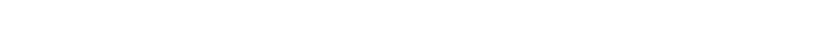# Get Started with HC-SR04, measure distance by ultrasound. Arduino code example

The HC-SR04 sensor allows you to make fairly precise distance measurements using an Arduino or a Raspberry Pi. In this tutorial we will learn how to use the sensor and perform distance measurements in a few lines of code using the Arduino IDE.

The HC-SR04 is an economical sensor available for less than 2€. The JSN SR04T is a waterproof version which may be suitable for level measurement in a water tank for example.

## Introduction to the HC-SR04 module

The HC-SR04 is a sensor that uses two digital signals. The first signal called Trig – Trigger – generates a beam of ultrasound. The ultrasound is reflected by the surface of the object (or the person) whose distance we want to know. This is the Echo signal that is recovered on the second pin of the sensor.

Knowing the time between the emission of the Trig signal and the reception Echo as well as the speed of propagation of the sound in the air we can deduce the distance using a small mathematical formula.

Distance = time / 2 * speed of sound in air

The measurement time is halved because the signal goes back and forth between the detector and the surface of the object.No need to try to re-develop the wheel, there are several Arduino and Python libraries to integrate the HC-SR04 into your projects. The easiest to use is the HCSR04 library developed by Martin Sosic.

Technical characteristics of HC-SR04

• Distance measuring range: 2cm to 450cm (4.5m)
• Measurement accuracy: 0.3cm
• Supply voltage: 5V
• Digital output: PWM
• Weight: 9g
• Dimensions: 45mm x 20mm x 18mm

## Necessary components

For this tutorial you will need the following material

## Circuit

Connect the four pins of the HC-SR04 to the Arduino using the following wiring diagram

 HC-SR04 Arduino VCC 5V GND GND Trig 2 Echo 3## Installation of the HCSR04 library

There are at least 7 libraries available directly from the Arduino IDE library manager. Here we will use the HCSR04 library developed by Martin Sosic.

Here are the different steps to install the HCSR04 library

1Start the Arduino IDE

2Open the library manager by following the Sketch path> Include a library> Manage libraries3Enter HCSR04 key word4Select the HCSR04 by Martin Sosic library and press the Install button5Close the library manager

This library contains two examples.

Simple allows to measure the distance in cm measured using the sensor and display it on the serial monitor.

Temperature provides superior measurement accuracy. The speed of sound varying according to the temperature of the medium, the calculation of the distance is compensated by taking this into account. We can retrieve the temperature using any sensor. DS18B20, DHT22, LM35 …

Some tutorials to get started with temperature measurement on Arduino

## Arduino code, compatible ESP32 and ESP8266

After installing the library, create a new file in File> New and paste the following code into the new sketch.

``````/*
Use the library HCSR04 by Martin Sosic
*/
#include <HCSR04.h>

// defines pins numbers
const int trigPin = 2;
const int echoPin = 3;

// Initialize sensor that uses digital pins trigPin and echoPin .
UltraSonicDistanceSensor distanceSensor(trigPin, echoPin);
void setup() {
// We initialize serial connection so that we could print values from sensor.
Serial.begin(9600);
}
void loop() {
// Every 500 miliseconds, do a measurement using the sensor and print the distance in centimeters.
Serial.println(distanceSensor.measureDistanceCm());
delay(500);
}
``````

## How the code works

Continue reading this section to learn how the code works, or skip ahead to the “Upload the program to the Arduino” section.

### Library

We start the program by declaring the HCSR04 library using the include command .

``#include <HCSR04.h>``

### Sensor pin

The two variables trigPin and echoPin are used to define the digital pins used by the sensor. You can assign any digital pin according to your need for other projects.

``````const int trigPin = 2;
``````const int echoPin = 3;
``````

### Sensor initialization

The sensor initialization is done by declaring a C++ object. The pins used by the sensor are indicated.

``UltraSonicDistanceSensor distanceSensor(trigPin, echoPin);``

### Setup()

The setup() function is executed only once when the Arduino board is powered on. Here, we will just need to start the serial link which will allow us to retrieve the sensor measurements from the Serial Monitor of the Arduino IDE.

``Serial.begin(9600);``

### Loop()

The loop() function runs ad infinitum. It only contains two lines of 2 codes.

The distanceSensor function which allows you to directly retrieve the distance in cm using the measureDistanceCm () method

``Serial.println(distanceSensor.measureDistanceCm());``

We slow down the execution of the loop loop while waiting for a certain delay. Here 500 milliseconds to return 2 measurements per second, which is already very fast.

``delay(500);``

## Upload the program to the Arduino

After creating the program, select the Arduino board you are using. Here an Arduino Uno by following the path Tools> Card type> Arduino Uno.Then select the USB port to which the card is connected by following the path Tools> Port.Open the serial monitor by choosing a baud rate of 9600 baud.## Demonstration

You should see the readings displayed on the serial monitor.Or as a graph using the Plotter

For further

Click to rate this post!
[Total: 0 Average: 0]